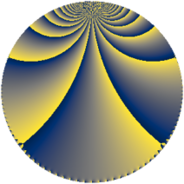# Properties

 Label 1344.4.bcLevel $1344$ Weight $4$ Character orbit 1344.bc Rep. character $\chi_{1344}(257,\cdot)$ Character field $\Q(\zeta_{6})$ Dimension $376$ Sturm bound $1024$

# Related objects

## Defining parameters

 Level: $$N$$ $$=$$ $$1344 = 2^{6} \cdot 3 \cdot 7$$ Weight: $$k$$ $$=$$ $$4$$ Character orbit: $$[\chi]$$ $$=$$ 1344.bc (of order $$6$$ and degree $$2$$) Character conductor: $$\operatorname{cond}(\chi)$$ $$=$$ $$21$$ Character field: $$\Q(\zeta_{6})$$ Sturm bound: $$1024$$

## Dimensions

The following table gives the dimensions of various subspaces of $$M_{4}(1344, [\chi])$$.

Total New Old
Modular forms 1584 392 1192
Cusp forms 1488 376 1112
Eisenstein series 96 16 80

## Trace form

 $$376q - 2q^{9} + O(q^{10})$$ $$376q - 2q^{9} + 58q^{21} - 4304q^{25} - 6q^{33} + 508q^{37} + 6q^{45} - 8q^{49} - 116q^{57} + 12q^{61} - 12q^{73} - 1234q^{81} - 984q^{85} + 110q^{93} + O(q^{100})$$

## Decomposition of $$S_{4}^{\mathrm{new}}(1344, [\chi])$$ into newform subspaces

The newforms in this space have not yet been added to the LMFDB.

## Decomposition of $$S_{4}^{\mathrm{old}}(1344, [\chi])$$ into lower level spaces

$$S_{4}^{\mathrm{old}}(1344, [\chi]) \cong$$ $$S_{4}^{\mathrm{new}}(21, [\chi])$$$$^{\oplus 7}$$$$\oplus$$$$S_{4}^{\mathrm{new}}(42, [\chi])$$$$^{\oplus 6}$$$$\oplus$$$$S_{4}^{\mathrm{new}}(84, [\chi])$$$$^{\oplus 5}$$$$\oplus$$$$S_{4}^{\mathrm{new}}(168, [\chi])$$$$^{\oplus 4}$$$$\oplus$$$$S_{4}^{\mathrm{new}}(336, [\chi])$$$$^{\oplus 3}$$$$\oplus$$$$S_{4}^{\mathrm{new}}(672, [\chi])$$$$^{\oplus 2}$$